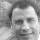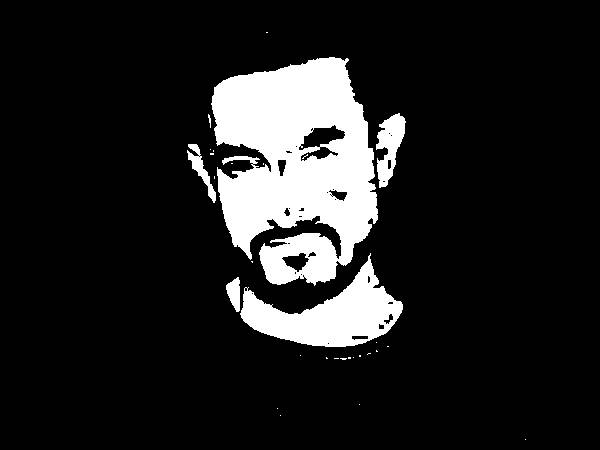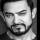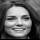The purpose of this project is to build an algorithm for detecting the gender of a person from the image of his/her face. The work-flow is as follows:

1. Build a reference set of face images
• 60 images of male faces
• 60 images of female faces
• Rescale all images to 40X40 pixels in grey values
1. Extract faces from test images
• 21 male faces
• 21 female faces
• Rescale all faces to 40X40 pixels in grey values
1. For each test image, compute the distance between the image and all reference images using Dynamic Time Warping (DTW)

2. Predict the gender based on which reference images the test image is closer to.

Note: The images are rescaled to size 40X40 in order to reduce the computational complexity of DTW distance computations which will be used for classification.

## Bulding The Reference Set

For reference, images of 60 male and 60 female faces (frontal view only) were taken from the Harvard Face Database.

Each image was in color and with .jpg extension. When we load them using jpeg package, they are loaded as arrays. The first two dimensions represent the row and columns of the pixed. The third dimesion contains the RGB values. The images were converted to grey by taking the average of RGB values for each pixel. Then they were rescaled to be 40X40 pixels. If the image has 160X120 pixels, then this image is rescaled by pre-multiplying a 40X160 matrix and post-multiplying a 120X40 matrix. These matix perform averaging of the blocks while scaling the original image to 40X40.

Reference : Baluja, S, Rowley, B Boosting Sex Identification Performance

library(jpeg)

# Rescale a face image to 40X40 size
Rescale4040 <- function(img){
dim_img <- dim(img)
img_grey <- matrix(data=0, nrow = dim_img, ncol = dim_img)

sapply(1:dim_img, function(i){
sapply(1:dim_img, function(j){
img_grey[i, j] <<- mean(img[i, j, ])
})
})

row_seq <- c(seq(1, dim_img, round(dim_img/40)), dim_img+1)
col_seq <- c(seq(1, dim_img, round(dim_img/40)), dim_img+1)

row_mult_matrix <- matrix(data=0, nrow=40, ncol=dim_img)

sapply(1:min(40, length(row_seq)-1), function(i){
if(row_seq[i] < dim_img){
row_mult_matrix[i,(row_seq[i]:min(row_seq[i+1]-1, dim_img))] <<- 1/(round(dim_img/40))
}

})

col_mult_matrix <- matrix(data=0, nrow=dim_img, ncol=40)

sapply(1:min(40, length(col_seq)-1), function(i){
if(col_seq[i] < dim_img){
col_mult_matrix[(col_seq[i]:min(col_seq[i+1]-1, dim_img)),i] <<- 1/(round(dim_img/40))
}

})

img_4040 <- row_mult_matrix %*% img_grey %*% col_mult_matrix

image_grey_4040 <- array(data=0, dim = c(40, 40, 3))

sapply(1:40, function(i){
sapply(1:40, function(j){
image_grey_4040[i, j, ] <<- img_4040[i, j]
})
})

return(image_grey_4040)
}

# Rescale all image files in the input_path folder and
# store the resultant image in output_path folder
RescaleAllFaces <- function(input_path, output_path){
raw_image_files <- list.files(input_path,
pattern = "*.jpg")

lapply(raw_image_files, function(file_name){
print(file_name)
img <- readJPEG(paste0(input_path, file_name))
curr_face <- Rescale4040(img)
print(paste0(output_path, strsplit(file_name, ".", fixed=T)[], "_4040.jpg"))
writeJPEG(curr_face,
paste0(output_path, strsplit(file_name, ".", fixed=T)[], "_4040.jpg"))
})

}

RescaleAllFaces('/Users/apple/Desktop/GenderDetection/faces_reference/males/',
'/Users/apple/Desktop/GenderDetection/faces_reference_40by40/males/')

RescaleAllFaces('/Users/apple/Desktop/GenderDetection/faces_reference/females/',
'/Users/apple/Desktop/GenderDetection/faces_reference_40by40/females/')

Shown below are the results of the above code on a particular input image file.input reference imageoutput reference image

## Buiding the Test set

The images of 21 males and 21 females (mostly celebrities) were taken from the internet. The images satisfied the following conditions.

1. There is only one face in the image.
2. The vetical central axis of the face was close to the central vertical column of the image.

### Extracting the face

The faces were extracted from the image using the following algorithm.

Reference: Gurel, C, Erden, A Face detection algorithm with facial feature extraction for face recognition system

#### White balancing

1. Calculate average value of red channel (Rav), green channel (Gav), and blue channel (Bav)
2. Calculate average gray, Grayav=(Rav+Gav+Bav)/3
3. Then, KR=Grayav/Rav, KG=Grayav/Gav, and KB=Grayav/Bav
4. Generate new image (NewI) from original image (OrjI) with RGB channel by New(R)=KR * Orj(R), New(G)=KG * Orj(G), and New(B)=KB * Orj(B)

#### Segmentation

Skin segmentation is applyied to mark regions that have a skin like color. The following condition must be satisfied for a pixel to be marked as skin pixel.

(r>95) & (g>40) & (b>20) & (max(r,g,b)-min(r,g,b)>15) & (|r-g|>15) & (r>g) & (r>b)

The pixels satisfying the above condition is marked as 1 and others as 0. This is done in both original and white balanced images. A logical AND operation is performed on the corresponding pixels of both images to get the final segmented image.

The results of segmentation on an image is shown below:input test imageoutput test segmented image

### Extracting the face

The following heuristic algorithm was used to extract the face region:

1. In the segmented image, search the centre columns between 35 and 65 % of the total number of columns. Fin the column that has maximum number of 1’s. Treat this column as the centre of the face.
2. The top margin of the face is the minimum row number that has the first 1 in the face centre column.
3. The bottom margin of the face is the maximum row number that has the last 1 in the face centre column.
4. The left margin of the face is the last column to the left of face centre that has at least 2% of the rows marked as 1’s.
5. The right margin of the face is the last column to the right of face centre that has at least 2% of the rows marked as 1’s.
6. If the face height (bottom margin - top margin) is more than 1.28 times the width (right margin - left margin), then reduce the height to 1.28 times the width by moving the bottom margin upwards.
7. Using the above 4 margins, exract the face from the orginal image.

The result of the above algorithm is shown below.output test image face extracted

### Rescaling the test faces

The faces of all 42 images were extracted using the above algorithm. Then they were rescaled to 40X40 pixels in the same manner as the reference images.

library(jpeg)

#White Balancing an image
WhiteBalance <- function(img){

dim_img <- dim(img)
img_wb <- array(data=0, dim = c(dim_img, dim_img, dim_img))

Rav <- mean(img[,,1])
Gav <- mean(img[,,2])
Bav <- mean(img[,,3])
Greyav <- (Rav + Gav + Bav)/3
Kr <- Greyav / Rav;
Kg <- Greyav / Gav;
Kb <- Greyav / Bav;
img_wb[,, 1] <- Kr * img[, , 1]
img_wb[,, 2] <- Kg * img[, , 2]
img_wb[,, 3] <- Kb * img[, , 3]

return(img_wb)
}

#Skin segmentation
DetectFace <- function(img){

img_wb <- WhiteBalance(img)

dim_img <- dim(img)

img_face_o <- array(data=0, dim = c(dim_img, dim_img, dim_img))
img_face <- array(data=0, dim = c(dim_img, dim_img, dim_img))

sapply(1:dim_img, function(i){
sapply(1:dim_img, function(j){
curr_rgb_o <- img[i, j, ]
curr_rgb <- img_wb[i,j,]

if((curr_rgb > 95/255) &
(curr_rgb > 40/255) &
(curr_rgb > 20/255) &
(max(curr_rgb)-min(curr_rgb) > 15/255) &
abs((curr_rgb-curr_rgb) > 15/255) &
(curr_rgb > curr_rgb) &
(curr_rgb > curr_rgb)){
img_face[i,j, ] <<- 1
}

if((curr_rgb_o > 95/255) &
(curr_rgb_o > 40/255) &
(curr_rgb_o > 20/255) &
(max(curr_rgb_o)-min(curr_rgb_o) > 15/255) &
abs((curr_rgb_o-curr_rgb_o) > 15/255) &
(curr_rgb_o > curr_rgb_o) &
(curr_rgb_o > curr_rgb_o)){
img_face_o[i,j, ] <<- 1
}

if (img_face_o[i,j, ] == 0 || img_face[i,j, ] == 0){
img_face[i,j, ] <<- 0
}

})
})

return(img_face)

}

#Extracting a face region from an image
ExtractFace <- function(img){
dim_img <- dim(img)
search_range <- c(floor(0.35*dim_img), floor(0.65*dim_img))
search_range <- c(floor(0.35*dim_img), floor(0.65*dim_img))

img_face_shaded <- DetectFace(img)

row_means <- apply(img_face_shaded[,,1], 2, mean)
img_centre <- search_range + which.max(row_means[search_range:search_range]) - 1

top_margin <- min(which(img_face_shaded[,img_centre,1]==1))
bottom_margin <- max(which(img_face_shaded[,img_centre,1]==1))
left_margin <- img_centre - min(which(row_means[img_centre:1] < 0.02))
right_margin <- img_centre + min(which(row_means[img_centre:dim_img] < 0.02))

if((bottom_margin - top_margin) > 1.28*(right_margin - left_margin)){
bottom_margin <- top_margin + floor(1.28 * (right_margin - left_margin))
}

print(c(top_margin, bottom_margin, left_margin, right_margin))
img_face <- img[top_margin:bottom_margin, left_margin:right_margin, ]

return(img_face)

}

# Extracting faces from image files in input_path and string the resultant image in output_path
ExtractAllFaces <- function(input_path, output_path){
raw_image_files <- list.files(input_path,
pattern = "*.jpg")

lapply(raw_image_files, function(file_name){
print(file_name)
img <- readJPEG(paste0(input_path, file_name))
curr_face <- ExtractFace(img)
print(paste0(output_path, strsplit(file_name, ".", fixed=T)[], "_face.jpg"))
writeJPEG(curr_face,
paste0(output_path, strsplit(file_name, ".", fixed=T)[], "_face.jpg"))
})

}

ExtractAllFaces('/Users/apple/Desktop/GenderDetection/raw_images/males/',
'/Users/apple/Desktop/GenderDetection/faces_test/males/')

ExtractAllFaces('/Users/apple/Desktop/GenderDetection/raw_images/females/',
'/Users/apple/Desktop/GenderDetection/faces_test/females/')

RescaleAllFaces('/Users/apple/Desktop/GenderDetection/faces_test/males/',
'/Users/apple/Desktop/GenderDetection/faces_test_40by40/males/')

RescaleAllFaces('/Users/apple/Desktop/GenderDetection/faces_test/females/',
'/Users/apple/Desktop/GenderDetection/faces_test_40by40/females/')

The examples of final test images are shown below.output test image maleoutput test image male

## Gender Prediction

The following algorithm was used to predict Gender

1. The male and female reference images were converted to 40X40 matrices with each element containing the grey value of the corresponding pixel.
2. The male and female test images were converted to 40X40 matrices with each element containing the grey value of the corresponding pixel.
3. For each test image:
• Multivariate Dynamic Time Warping was used to determine the distance between the image and all 120 reference images. Manhattan Distance is used as a distance metric.
• The closest 10 male reference images and closest 10 female reference images are selected. If the average distance from male reference images in less than the average distance from female reference images, the test image is calssified as male, otherwise female.
options(warn=-1)
library(dtw)
library(jpeg)
library(data.table)

#Convert image to matrices
ImageAvg <- function(img){
dim_img <- dim(img)
img_grey <- matrix(data=0, nrow = dim_img, ncol = dim_img)

sapply(1:dim_img, function(i){
sapply(1:dim_img, function(j){
img_grey[i, j] <<- mean(img[i, j, ])
})
})
return(img_grey)
}

ImageAvgAll <- function(input_path){

raw_image_files <- list.files(input_path,
pattern = "*.jpg")

res <- list()

sapply(1:length(raw_image_files), function(i){
file_name <- raw_image_files[[i]]
print(file_name)
img <- readJPEG(paste0(input_path, file_name))
curr_avg <- ImageAvg(img)
res[[i]] <<- curr_avg

})

return(res)

}

males_image_avg_train <- ImageAvgAll('/Users/apple/Desktop/GenderDetection/faces_reference_40by40/males/')
females_image_avg_train <- ImageAvgAll('/Users/apple/Desktop/GenderDetection/faces_reference_40by40/females/')

test_image_files_males <- list.files('/Users/apple/Desktop/GenderDetection/faces_test_40by40/males/')
test_image_files_females <- list.files('/Users/apple/Desktop/GenderDetection/faces_test_40by40/females/')

test_male_dist <- do.call('rbind',
lapply(test_image_files_males, function(filename){
curr_avg <- ImageAvg(readJPEG(paste0('/Users/apple/Desktop/GenderDetection/faces_test_40by40/males/',
filename)))

curr_dist_male <- sapply(1:length(males_image_avg_train), function(i){
curr_dtw <- dtw(curr_avg, males_image_avg_train[[i]],
dist.method='Manhattan')
return(curr_dtw$distance) }) curr_dist_female <- sapply(1:length(females_image_avg_train), function(i){ curr_dtw <- dtw(curr_avg, females_image_avg_train[[i]], dist.method='Manhattan') return(curr_dtw$distance)
})

curr_avg_male_dist <- mean(sort(curr_dist_male)[1:10])
curr_avg_female_dist <- mean(sort(curr_dist_female)[1:10])

curr_dt <- data.table(filename = filename,
avg_male_dist = curr_avg_male_dist,
avg_female_dist = curr_avg_female_dist,
pred = ifelse(curr_avg_male_dist < curr_avg_female_dist,
'male',
'female'))
return(curr_dt)
}))

test_female_dist <- do.call('rbind',
lapply(test_image_files_females, function(filename){
curr_avg <- ImageAvg(readJPEG(paste0('/Users/apple/Desktop/GenderDetection/faces_test_40by40/females/',
filename)))

curr_dist_male <- sapply(1:length(males_image_avg_train), function(i){
curr_dtw <- dtw(curr_avg, males_image_avg_train[[i]],
dist.method='Manhattan')
return(curr_dtw$distance) }) curr_dist_female <- sapply(1:length(females_image_avg_train), function(i){ curr_dtw <- dtw(curr_avg, females_image_avg_train[[i]], dist.method='Manhattan') return(curr_dtw$distance)
})

curr_avg_male_dist <- mean(sort(curr_dist_male)[1:10])
curr_avg_female_dist <- mean(sort(curr_dist_female)[1:10])

curr_dt <- data.table(filename = filename,
avg_male_dist = curr_avg_male_dist,
avg_female_dist = curr_avg_female_dist,
pred = ifelse(curr_avg_male_dist < curr_avg_female_dist,
'male',
'female'))
return(curr_dt)
}))

## Results

The overall accuracy of gender prediction on 42 test images was 69%. On male samples, the accuracy was 76% and on female samples the accuracy was 62%

Gender Prediction On Male Faces
pred N
male 16
female 5
Gender Prediction On Female Faces
pred N
female 13
male 8

The lower accuracy of females can probably be explained by the larger diversity in female faces.

## Conclusion

Since this was an experiment with very small number of reference images was used, nearly 70% accuracy is very encouraging.